# Basic Image Noise and Filter Tweaks

Image Noise and Filter

# Basic Image Manipulations¶

In :
# Load packagesimport osfrom PIL import Image, ImageFilterimport numpy as npimport matplotlib.pyplot as pltfrom scipy.ndimage import filters# Ensure plots embeded in notebook%matplotlib inline
In :
# Open original imageimg = Image.open('ducks.jpg','r')# Cannot use Image show directly#img.show()   # Image will show in external window# Use numpy array, convert to gray scalingim = np.array(img.convert('L'))# Matplotlib doesn't support bmp natively# Need to change color map to grayscale and set vmin, vmax to reproduce the same luminace of originalplt.imshow(im, cmap='gray',vmin=0, vmax=255) plt.show()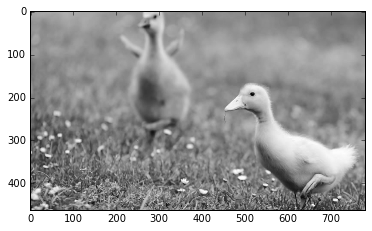In :
# Add Noise (Gaussian)noise_sigma = 30noise = noise_sigma * np.random.randn(*im.shape)# the histogram of the noiseplt.subplot(1,2,1)n, bins, patches = plt.hist(noise,20)# show the ducks with noisenoisy_ducks = im + noise;plt.subplot(1,2,2)plt.imshow(noisy_ducks, cmap='gray', vmin=0, vmax=255)plt.show()# Left shows noise is a normal distribution# Right shows the image with noise added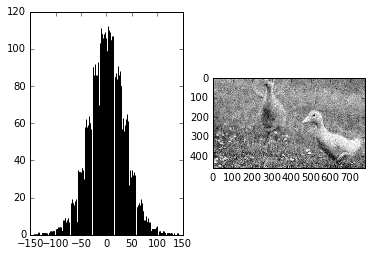In :
# Apply Gaussian smoothing kernal to noisy imagefilter_sigma = 3smooth_ducks = filters.gaussian_filter(noisy_ducks,filter_sigma)plt.imshow(smooth_ducks, cmap='gray', vmin=0, vmax=255)plt.show()# Noisy image becomes smooth, or blurred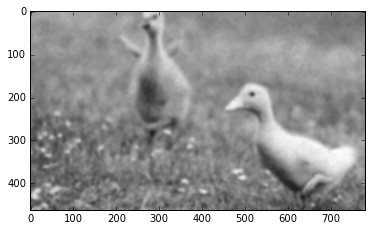In :
# Sharpen the blurred image 1: Using PIL built in filtersharp_img = Image.fromarray(np.uint8(smooth_ducks)).filter(ImageFilter.SHARPEN)plt.imshow(sharp_img, cmap='gray', vmin=0, vmax=255)plt.show()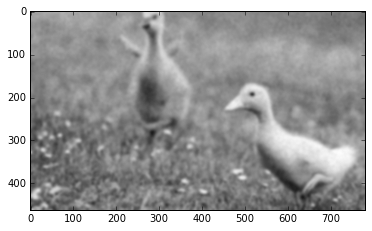In :
# Sharpen the blurred image 2: using custom filter# Multiplying each piexl by 2, then subtracting average value of neighbors (bluring filter)# Overall, the impact is a sharpening filter ("unsharp mark", a term come from old days with dark room)# It accenturates the difference with local average# This section needs opencv installed, which mine does not right now, skipped for now
In :
# Sharpen the blurred image 3: using custom filter# Similar to the above one, but directly use gaussian filter twice to create similar effectalpha = 30filter_blurred_image = filters.gaussian_filter(smooth_ducks, 1)sharp_img_3 = smooth_ducks + alpha * (smooth_ducks - filter_blurred_image)plt.imshow(sharp_img_3, cmap='gray', vmin=0, vmax=255)plt.show()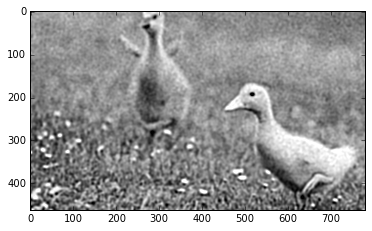In [ ]:


Published: September 08 2016

• category:
• tags: